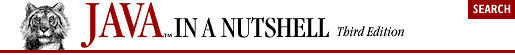## 4.14. Cryptography

The java.security package includes cryptography-based classes, but it does not contain classes for actual encryption and decryption. That is the job of the javax.crypto package. This package supports symmetric-key cryptography, in which the same key is used for both encryption and decryption and must be known by both the sender and the receiver of encrypted data. The SecretKey interface represents an encryption key; the first step of any cryptographic operation is to obtain an appropriate SecretKey. Unfortunately, the keytool program supplied with the Java SDK cannot generate and store secret keys, so a program must handle these tasks itself. Here is some code that shows various ways to work with SecretKey objects:

```import javax.crypto.*;
import javax.crypto.spec.*;

// Generate encryption keys with a KeyGenerator object
KeyGenerator desGen = KeyGenerator.getInstance("DES");       // DES algorithm
SecretKey desKey = desGen.generateKey();                     // Generate a key
KeyGenerator desEdeGen = KeyGenerator.getInstance("DESede"); // Triple DES
SecretKey desEdeKey = desEdeGen.generateKey();               // Generate a key

// SecretKey is an opaque representation of a key. Use SecretKeyFactory to
// convert to a transparent representation that can be manipulated: saved
// to a file, securely transmitted to a receiving party, etc.
SecretKeyFactory desFactory = SecretKeyFactory.getInstance("DES");
DESKeySpec desSpec = (DESKeySpec)
desFactory.getKeySpec(desKey, javax.crypto.spec.DESKeySpec.class);
byte[] rawDesKey = desSpec.getKey();
// Do the same for a DESede key
SecretKeyFactory desEdeFactory = SecretKeyFactory.getInstance("DESede");
DESedeKeySpec desEdeSpec = (DESedeKeySpec)
desEdeFactory.getKeySpec(desEdeKey, javax.crypto.spec.DESedeKeySpec.class);
byte[] rawDesEdeKey = desEdeSpec.getKey();

// Convert the raw bytes of a key back to a SecretKey object
DESedeKeySpec keyspec = new DESedeKeySpec(rawDesEdeKey);
SecretKey k = desEdeFactory.generateSecret(keyspec);

// For DES and DESede keys, there is an even easier way to create keys
// SecretKeySpec implements SecretKey, so use it to represent these keys
byte[] desKeyData = new byte;        // Read 8 bytes of data from a file
byte[] tripleDesKeyData = new byte; // Read 24 bytes of data from a file
SecretKey myDesKey = new SecretKeySpec(desKeyData, "DES");
SecretKey myTripleDesKey = new SecretKeySpec(tripleDesKeyData, "DESede");```

Once you have obtained an appropriate SecretKey object, the central class for encryption and decryption is Cipher. Use it like this:

```SecretKey key;     // Obtain a SecretKey as shown earlier
byte[] plaintext;  // The data to encrypt; initialized elsewhere

// Obtain an object to perform encryption or decryption
Cipher cipher = Cipher.getInstance("DESede");  // Triple-DES encryption
// Initialize the cipher object for encryption
cipher.init(Cipher.ENCRYPT_MODE, key);
// Now encrypt data
byte[] ciphertext = cipher.doFinal(plaintext);

// If we had multiple chunks of data to encrypt, we can do this
cipher.update(message1);
cipher.update(message2);
byte[] ciphertext = cipher.doFinal();

// We simply reverse things to decrypt
cipher.init(Cipher.DECRYPT_MODE, key);
byte[] decryptedMessage = cipher.doFinal(ciphertext);

// To decrypt multiple chunks of data
byte[] decrypted1 = cipher.update(ciphertext1);
byte[] decrypted2 = cipher.update(ciphertext2);
byte[] decrypted3 = cipher.doFinal(ciphertext3);```

The Cipher class can also be used with CipherInputStream or CipherOutputStream to encrypt or decrypt while reading or writing streaming data:

```byte[] data;                              // The data to encrypt
SecretKey key;                            // Initialize as shown earlier
Cipher c = Cipher.getInstance("DESede");  // The object to perform encryption
c.init(Cipher.ENCRYPT_MODE, key);         // Initialize it

// Create a stream to write bytes to a file
FileOutputStream fos = new FileOutputStream("encrypted.data");

// Create a stream that encrypts bytes before sending them to that stream
CipherOutputStream cos = new CipherOutputStream(fos, c);

cos.write(data);                      // Encrypt and write the data to the file
cos.close();                          // Always remember to close streams
java.util.Arrays.fill(data, (byte)0); // Erase the unencrypted data```

Finally, the javax.crypto.SealedObject class provides an especially easy way to perform encryption. This class serializes a specified object and encrypts the resulting stream of bytes. The SealedObject can then be serialized itself and transmitted to a recipient. The recipient is only able to retrieve the original object if she knows the required SecretKey:

```Serializable o;           // The object to be encrypted; must be Serializable
SecretKey key;                              // The key to encrypt it with
Cipher c = Cipher.getInstance("Blowfish");  // Object to perform encryption
c.init(Cipher.ENCRYPT_MODE, key);           // Initialize it with the key
SealedObject so = new SealedObject(o, c);   // Create the sealed object

// Object so is a wrapper around an encrypted form of the original object o;
// it can now be serialized and transmitted to another party.
// Here's how the recipient decrypts the original object
Object original = so.getObject(key);        // Must use the same SecretKey```